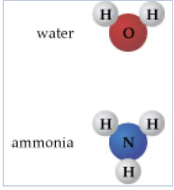# Problem: Part B. How many atoms of hydrogen (H) are present in 200 molecules of ammonia (NH3)?Express your answer numerically.Using mass measurements, early chemists were able to determine that for pure substances, the mass proportions of the composite elements were the same, no matter how much of the substance was analyzed. This became known as the law of definite proportions, or the law of constant composition. Dalton interpreted that this constant mass composition arose from constant composition of atoms. Working with this concept will help you to better understand the particulate nature of matter. To answer the questions, refer to the structures of water and ammonia shown here.

###### FREE Expert SolutionView Complete Written Solution
###### Problem Details

Part B. How many atoms of hydrogen (H) are present in 200 molecules of ammonia (NH3)?